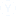Ques. 1992 Feb have 5 Sundays. In which year, the month Feb have 5 Sundays next?1-1 of  1
• Explanation:
If in 1992, February has 5 Sundays, it means 29 February 1992 = Sunday
Each year has one odd day.. i.e. mod(365,7) = 1
For the next February to have 5 Sundays, it should be a leap year and the sum of odd numbers to that day should be a multiple of 7
Odd days from 1993 till 1996 (next leap year)  are 1, 1, 1, 2 . Sum = 5, not a multiple of 7
Odd days from 1993 to 2000 are 5 (previous), 1, 1, 1, 2. Sum = 5+5 = 10, not a multiple of 7
Till 2004, Sum = 15, not a multiple of 7
Till 2008, Sum = 20, not a multiple of 7
Till 2012, Sum = 25, not a multiple of 7
Till 2016, Sum = 30, not a multiple of 7
Till 2020, Sum = 35, a multiple of 7Other Related Discussions